Next: Exercises Up: Wave Propagation in Inhomogeneous Previous: WKB Reflection Coefficient

# Jeffries Connection Formula

In the preceding section, there is a tacit assumption that the square of the refractive index,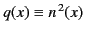, is a real function. However, as is apparent from Equation (1056), this is only the case in the ionosphere as long as electron collisions are negligible. Let us generalize our analysis to take electron collisions into account. In fact, the main effect of electron collisions is to move the zero of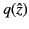a short distance off the real axis (the distance is relatively short provided that we adopt the physical ordering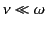). The arrangement of Stokes and anti-Stokes lines around the new zero point, located at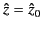, is sketched in Figure 25. Note that electron collisions only significantly modify the form ofin the immediate vicinity of the zero point. Thus, sufficiently far away fromin the complex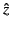-plane, the WKB solutions, as well as the locations of the Stokes and anti-Stokes lines, are exactly the same as in the preceding section.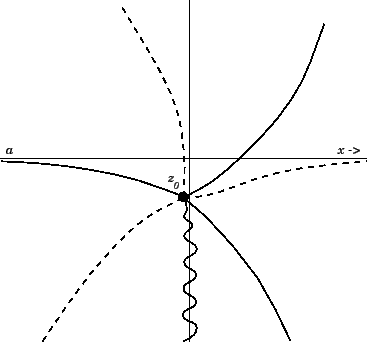The WKB solutions (1199) and (1200) are valid all the way along the real axis, except for a small region close to the origin where electron collisions significantly modify the form of. Thus, we can still adopt the physically reasonable decaying solution (1201) on the positive real axis. Let us trace this solution in the complex-plane until we reach the negative real axis. We can achieve this by moving in a semi-circle in the upper half-plane. Because we never move out of the region in which the WKB solutions (1199) and (1200) are valid, we conclude, by analogy with the preceding section, that the solution on the negative real axis is given by Equation (1209). Of course, in all of the WKB solutions the point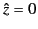must be replaced by the new zero point. The new formula for the reflection coefficient, which is just a straightforward generalization of Equation (1213), is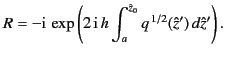(1212)

This is called the Jeffries connection formula, after H. Jeffries, who discovered it in 1923. Thus, the general expression for the reflection coefficient is incredibly simple. We just integrate the WKB solution in the complex-plane from the phase reference level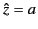to the zero point, square the result, and multiply by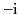. Note that the path of integration betweenanddoes not matter, because of Cauchy's theorem. Note, also, that because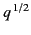is, in general, complex along the path of integration, we no longer have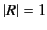. In fact, it is easily demonstrated that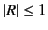. Thus, when electron collisions are included in the analysis, we no longer obtain perfect reflection of radio waves from the ionosphere. Instead, some (small) fraction of the radio energy is absorbed at each reflection event. This energy is ultimately transferred to the particles in the ionosphere with which the electrons collide.Next: Exercises Up: Wave Propagation in Inhomogeneous Previous: WKB Reflection Coefficient
Richard Fitzpatrick 2014-06-27